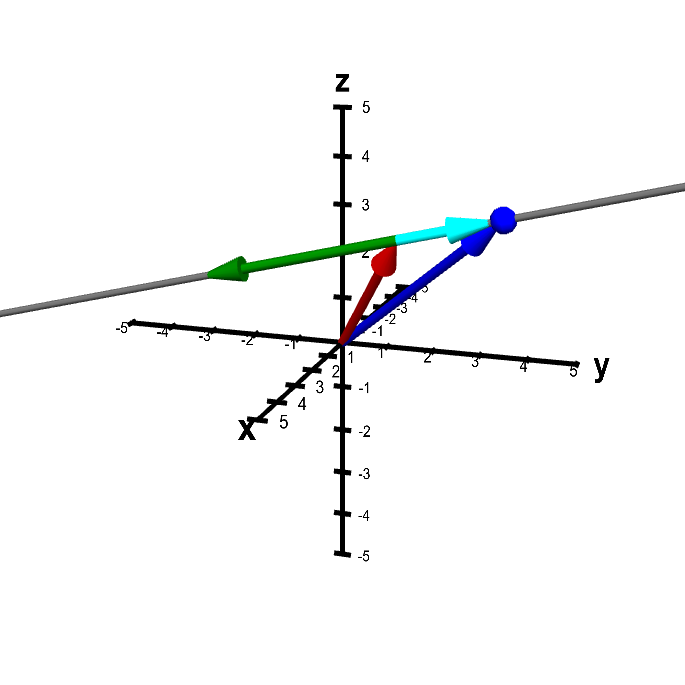# Math Insight

### Applet: A point on the line determined by two vectorsThe point $\color{blue}{\vc{x}}$ (represented by the blue point as well as the blue vector) is constrained to be on the line passing through the point $\color{red}{\vc{a}}$ (represented by the red vector) and parallel to the vector $\color{green}{\vc{v}}$ (in green). You can move $\color{blue}{\vc{x}}$ by dragging it with the mouse, but it is constrained to be on the line. You can change the line by dragging $\color{red}{\vc{a}}$ or $\color{green}{\vc{v}}$ with the mouse.

Since $\color{blue}{\vc{x}}$ is on the line, the vector $\color{green}{\vc{v}}$ is always parallel to the vector $\color{cyan}{\vc{x}-\vc{a}}$ (in cyan).